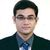Announcements
Product Release Webinar: Qlik Insider airing December 6! REGISTER TODAY!
cancel
Showing results for
Did you mean:Not applicable

## Show trend when second value is zero

Hello,

This is a newbish questions,

But if i wanna show a trend based on two values i divied them,

So eg i have:

sum(amount) / sum(amount2)

Lets say

Sum(amount) = 1 and sum(amount2) = 0

Then i want a trend which says its 100% , but as everybody knows, you cannot divied by zero.

What can i do?

1 Solution

Accepted SolutionsHi,

its wrong cause

Sum({<CW={1}>} TotalRows) / sum({<PW={1}>} TotalRows) will never be zero when its 1/0.

Sum({<CW={1}>} TotalRows) / if(sum({<PW={1}>} TotalRows)=0,1,sum({<PW={1}>} TotalRows))

Regards,

Kaushik Solanki

Regards,
Kaushik
5 RepliesHi,

You can use the if statement to check whether the sum(Amount2) is 0 or not, if its zero then replace it by 1.

in that case 1/1 will be 100%

Regards,

Kaushik Solanki

Regards,
KaushikNot applicable
Author

I already tried that, but i cannot seem to get it to work then?

I tried

=if(Sum({<CW={1}>} TotalRows) / sum({<PW={1}>} TotalRows) = 0,1,Sum({<CW={1}>} TotalRows) / sum({<PW={1}>} TotalRows))

However i might did something wrong?Specialist

Use like this

=If(Sum(amount2) > 0, Sum(amount) / Sum(amount2), Sum(amount))

Regards,Hi,

its wrong cause

Sum({<CW={1}>} TotalRows) / sum({<PW={1}>} TotalRows) will never be zero when its 1/0.

Sum({<CW={1}>} TotalRows) / if(sum({<PW={1}>} TotalRows)=0,1,sum({<PW={1}>} TotalRows))

Regards,

Kaushik Solanki

Regards,
Kaushik